# Module 7 - Residuals Diagnosis

In modules five and six, we demonstrated the time series modeling procedure from model specification to calibration.

In this module, we will look into the model’s residuals time series and examine the underlying model assumptions (e.g. normality, etc.).

In a nutshell, a time series model draws some patterns for the evolution of values over time and assumes the error terms (i.e. residuals) to be independent and following particular probability distribution. Once we fit the sample data into the model, it is imperative to examine those residuals for independence and whether their values follow the assumed distribution.

Example 1: An ARMA(p,q) model assumes the residuals time series to be a Gaussian white-noise distribution.

$$(1-\sum_{i=1}^p\phi_i L^i)y_t=(1+\sum_{j=1}^q \theta_j L^j)a_t$$ $$a_t \sim \mathrm{i.i.d}\sim \Phi(0,\sigma^2)$$

Example 2: A GARCH(1,1) model assumes the standardized residuals to be Gaussian white-noise with zero mean and unity variance.

$$y_t=\mu + a_t$$ $$\sigma_t^2=\alpha_o + \alpha_1 a_{t-1}^2 + \beta_1 \sigma_{t-1}^2$$ $$a_t = \epsilon_t \times \sigma_t$$ $$\epsilon_t \sim \mathrm{i.i.d} \sim \Phi(0,1)$$

To avoid confusion as to when you should use the regular residuals $\{a_t\}$ or the standardized residuals $\{\epsilon_t\}$, we will limit ourselves to the standardized residuals. This simplifies the diagnosis dramatically:

$$\epsilon_t \sim \mathrm{i.i.d} \sim \Phi(0,1)$$

Why do we care? The objective of a time series is forecasting, so by ensuring that our model properly fits the data and meets all assumptions, we can have faith in the projected forecast.

Note: similar to modules 5 and 6, we will be using the S&P 500 weekly log returns time series between Jan 2009 and July 2012.

NumXL supports numerous functions to help us construct residuals series and conduct statistical tests to answer the independence/probability distribution questions.

In this module, you don’t need to launch any wizard or create any formula; all the tests we need are included in the model table (right-most part):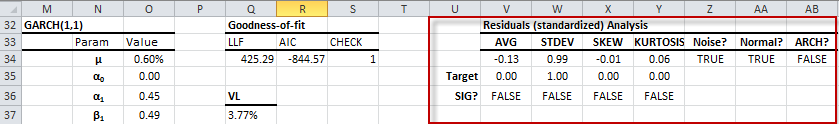The standardized residuals diagnosis includes the following hypothesis testings:

1. Population mean ($H_o : \mu = 0$)
2. Population standard deviation ($H_o : \sigma = 1$)
3. Population skew ($H_o : S = 0$)
4. Population excess-kurtosis ($H_o : K = 0$)
5. White-noise or serial-correlation test ($H_o : \rho_1=\rho_2=\cdots = 0$)
6. Normality test
7. ARCH effect test

The first four tests examine the distribution center, dispersion, symmetry, and far-end tails. The Normality test complements these tests by assuming a specific distribution – Gaussian.

$$\epsilon_t \sim \Phi(0,1)$$

The white-noise and ARCH effect tests address a different concern: independence of residual observations.

$$\epsilon_t \sim \mathrm{i.i.d}$$

Since the independence test is quite a complex topic, we simplify it by examining the linear and quadratic order dependency.

Let’s analyze the residuals diagnosis table results:

1. The population mean (i.e. AVG) test shows that the sample average is not significantly different from zero (Target). As a result, the residuals’ distribution has a mean of zero.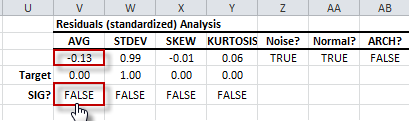2. The population standard deviation (i.e. STDEV) test shows that sample data standard deviation is not significantly different from one (1).3. The population skew test shows that the sample skew is not significantly different from zero. The residuals distribution is symmetrical.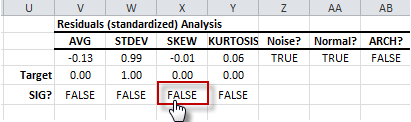4. The population excess kurtosis test indicates sample kurtosis is not significantly different from that of a normal distribution (i.e. excess kurtosis = 0). The residuals distribution tails are normal.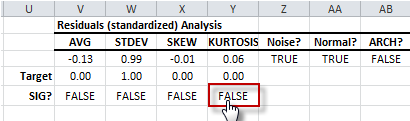5. So far, the distribution of the residuals seems like a Gaussian distribution. The Normality test shows that standardized residuals are likely to be sampled from a normal population.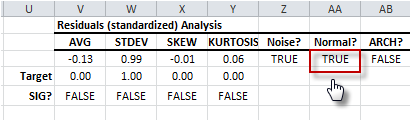6. Now let’s examine the interdependence concern among the values of the residuals. First, let’s examine the first-order dependence (linear) or serial correlation using the white-noise test (Ljung-Box). The test shows no sign of significant serial correlation.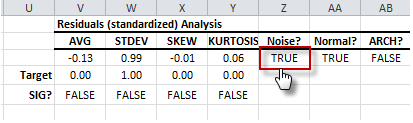7. Let’s examine the second-order dependence (quadratic) or ARCH effect. The ARCH effect test shows an insignificant serial correlation in the squared residuals or the absence of an ARCH effect.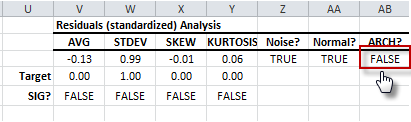As a result, the standardized residuals are independent and identically Gaussian distributed. Thus, the GARCH model assumption is met. The model is fair.

Let’s plot the distribution of the standardized residuals and the QQ-Plot.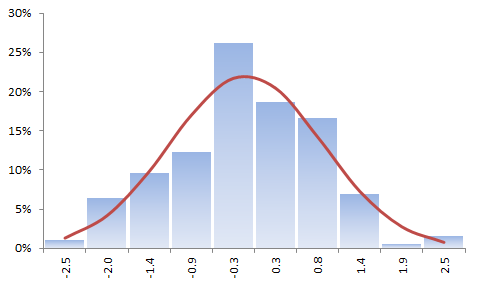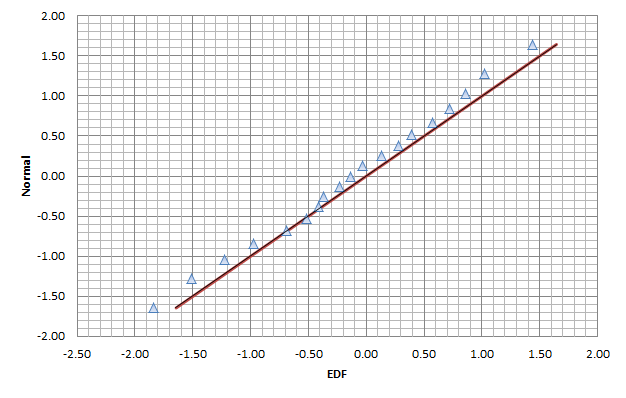The sample data histogram does not strongly imply a Gaussian distribution, but this is due to the construct of the histogram as a rough estimate of the underlying distribution.

On the other hand, the QQ-plot confirms our earlier finding of normality and an absence of fat-tails on either end.